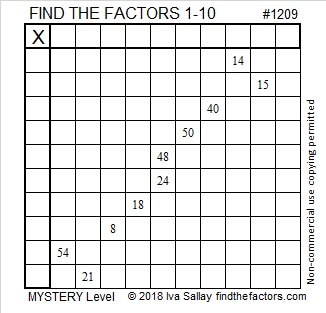# 1209 Mystery Level

How easy or difficult is this mystery level puzzle? That’s part of the mystery! Once you solve it, you will know, and you don’t have to tell let anybody else know.Print the puzzles or type the solution in this excel file: 10-factors-1199-1210

• 1209 is a composite number.
• Prime factorization: 1209 = 3 × 13 × 31
• The exponents in the prime factorization are 1, 1, and 1. Adding one to each and multiplying we get (1 + 1)(1 + 1)(1 + 1) = 2 × 2 × 2 = 8. Therefore 1209 has exactly 8 factors.
• Factors of 1209: 1, 3, 13, 31, 39, 93, 403, 1209
• Factor pairs: 1209 = 1 × 1209, 3 × 403, 13 × 93, or 31 × 39
• 1209 has no square factors that allow its square root to be simplified. √1209 ≈ 34.7707Did you notice the pattern in the factors?  3×13×31 = 1209
39 and 93 are two of its factors, as well!

1209 is also the hypotenuse of a Pythagorean triple:
465-1116-1209 which is (5-12-13) times 93

This site uses Akismet to reduce spam. Learn how your comment data is processed.